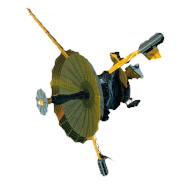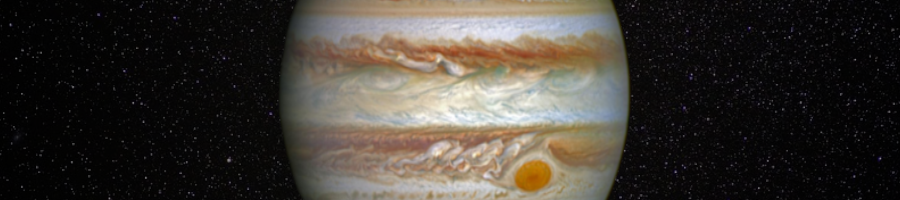GALILEO

## Galileo EPD Handbook

### Chapter 1. Instrument Summary

#### CMS Subsystem (Pre-Challenger Information)

Source: JOMPI Proposal, November 1976

The CMS is a three parameter (time-of-flight, dE/dx and E) solid state detector telescope designed to measure with high resolution the elemental composition, energy spectra and pitch angle distribution of the Jovian magnetospheric Z>=2 energetic particle environment throughout the Orbiter mission.  The telescope consists of a mosaic of three very thin front elements (2 and 5 micron thick totally depleted surface barrier detectors) that are separated by a 7.5 cm time of flight path from the rear detectors. Incident particle energy loss (dE/dx) is measured in the front element, particle time-of-flight (TOF) is measured between the front and rear detectors, and residual energy is measured in the rear detector. Three parameter analysis is thus performed on particles penetrating the front detector, providing accurate elemental resolution with high immunity to accidental events over an energy range (for Na ions, for example) from H100 keV/nuc to >10 MeV/nuc. Single parameter rate channels extend coverage for medium-Z nuclei to 20 to 50 keV/nuc, and unique two and three parameter particle identifier circuits, based on logarithmic amplifiers, provide rate data and priority information with excellent temporal and angular resolution. An adaptive electronic priority system (utilizing the particle group identifier channels) establishes priority for full three-parameter analysis on the basis of atomic number, thus assuring adequate sampling for all species, over the full range of nuclear charge and energy. These proven design factors, plus a low energy threshold, a good geometry factor (total G = 0.01 cm2 ster. for three-parameter analysis and = 0.31 cm2 ster. for single parameter analysis), and wide dynamic range allow the CMS to accurately analyze the composition of the Jovian radiation environment from the low fluxes expected at apojove and off the equatorial plane to the regions of intense flux at the orbits of Io and Ganymede.

#### Geometrical Factors

Source: Ted Fritz, SEL Memo, April 24, 1978

During the March 15-21 meetings held at MPAE I was given the action item of calculating the geometric factor of the CMS telescope design as schematically outlined by Herr Bolme. Upon returning from Germany I discussed the problem with Harold Leinbach, and he agreed to use his computer program to make these calculations. The resultant geometric factor for the front elements is:

 Detector Area (mm2) g (cm2 ster.) Max θ/Z* Ja 25 0.1044 28 degrees Jb 25 0.1044 28 degrees Jc 5 0.0211 24.5 degrees Total J (single parameter) 0.2299

Geometric factor of detector K (100 mm2).

 Through detector g (cm2 ster.) Max θ/Z** Ja 0.00497 6.8 degrees Jb 0.00497 6.8 degrees Jc 0.00099 5.5 degrees Total K (coincidence) 0.01093

*This angle represents the largest possible half-angle at which a particle still strikes the J detector, relative to the axis of the collimator.

**This angle represents the largest possible angle at which a particle still strikes the K detector after passing through the respective J detector, relative to an axis through detectors Ja, Jb, or Jc.

These numbers are in reasonable agreement with the proposal values of 0.31 cm2 ster. (single parameter) and 0.01 cm2 ster. (coincidence).

Note on Geometric Factor for 0 Degree End in CMS Unit

Source: B. Wilkin, MPAE, July 24, 1979

The 5μ/50 mm2 telescope in the ΔE/E-telescopes is designed with a 45º opening angle centered on the surface of the back detector. The total collimator length of 21 mm (from surface of front detector) defines then a collimator angle of β = 18.5º. (Any growth of the collimator length l will be done with β = 18.5 = const.) The collimator ratio is presently l/r = 5.4. (According to T. Mueller it can be increased to 6.0.)

The differential geometric factor dG/dα is shown in Figure 1-13 as a function of the angle α (curve (2)). (α denotes the angle of incidence formed by a parallel beam with the collimator center line; compare Figure 1-14).  In Figure 1-13 the effect of the collimator is described by curve (3), curve (1) is the dependence of dG/dα with no collimator at all. The function dG/dα reaches the maximum value (modal value) at an angle somewhat larger than the collimator angle β = 18.5º. α(mode) = 200 dG/dα drops to zero for the maximum angle accepted by the collimator αgr=dG/dα drops to zero for the maximum angle accepted by the collimator αgr = 35º. (Note: Figures 1-13 and 1-14 are not available.)

It turns out that the median value am for the distribution dG/dα is pretty close to β: αm = 18.5º. The median value of the detector thickness corresponding to αm is then: dm = 1.054 d. If we define a FWHM value for the distribution dG/dα we find FWHM - 20.5º corresponding to an effective range for dm: dm = (1.054 +/- 0.05) d, i.e., the effective thickness of the detector is increased by 5%. The maximum thickness occurs for a beam with α=αgr=35º: dmax = 1.225 d.

Figure 1-15 shows the effect of the thickness variation in the ΔE/E curves of a 5μ detector. The FWHM variation seems to be comparable with the other statistical fluctuations in the signal. This effect seems to be acceptable. The upper extreme limit, on the other hand, is quite large and may in fact generate some minor overlap. However, dmax occurs only with a very small probability. (Note: Figure 1-15 is not available.)

Conclusion: The large opening in the CMS ΔE/E telescopes seems to be an adequate compromise if a large geometric factor is the more important feature. Some additional spread is to be expected from the large range permitted for the angle α. The FWHM fluctuation introduced by thickness variations seems to be comparable with contributions from other noise sources in the ΔE/E system.

According to T. Mueller, the total length of the CMS unit can be increased to 150 mm. As a result we modified the backlooking (dual telescope) collimator as follows:

l = 300 mm (surface front det.-edge of collimator)
β = 16.74º
αmax = 22.5º
αgr = 30º

The angles are somewhat smaller than before and this will reduce the signal spread introduced by thickness variations.